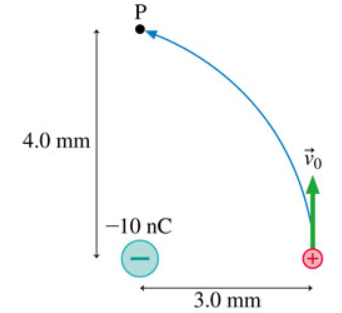# Problem: A proton follows the path shown in (Figure 1) . Its initial speed is v0 = 2.9×106 m/s. What is the proton's speed as it passes through point P? Express your answer to two significant figures and include the appropriate units.

###### FREE Expert Solution

Electrostatic potential energy:

$\overline{){\mathbf{U}}{\mathbf{=}}\frac{\mathbf{k}{\mathbf{q}}_{\mathbf{1}}{\mathbf{q}}_{\mathbf{2}}}{\mathbf{r}}}$

Law of conservation of energy:

$\overline{){{\mathbf{K}}}_{{\mathbf{i}}}{\mathbf{+}}{{\mathbf{U}}}_{{\mathbf{i}}}{\mathbf{+}}{{\mathbf{W}}}_{{\mathbf{nc}}}{\mathbf{=}}{{\mathbf{K}}}_{{\mathbf{f}}}{\mathbf{+}}{{\mathbf{U}}}_{{\mathbf{f}}}}$ Wnc is the work done by non-conservative forces like friction.

Ki + Ui + Wnc = Kf + Uf

(1/2)mv02 + kq1q2/ri + 0 = (1/2)mvf2 + kq1q2/rf

81% (350 ratings)###### Problem DetailsA proton follows the path shown in (Figure 1) . Its initial speed is v0 = 2.9×106 m/s. What is the proton's speed as it passes through point P? Express your answer to two significant figures and include the appropriate units.